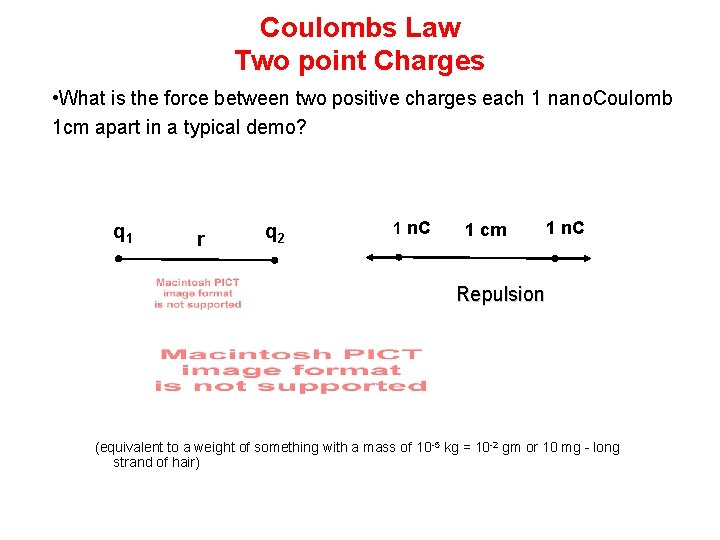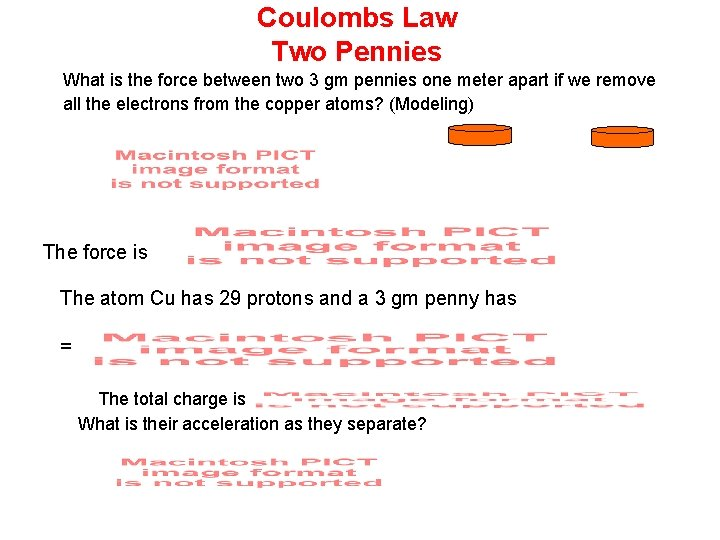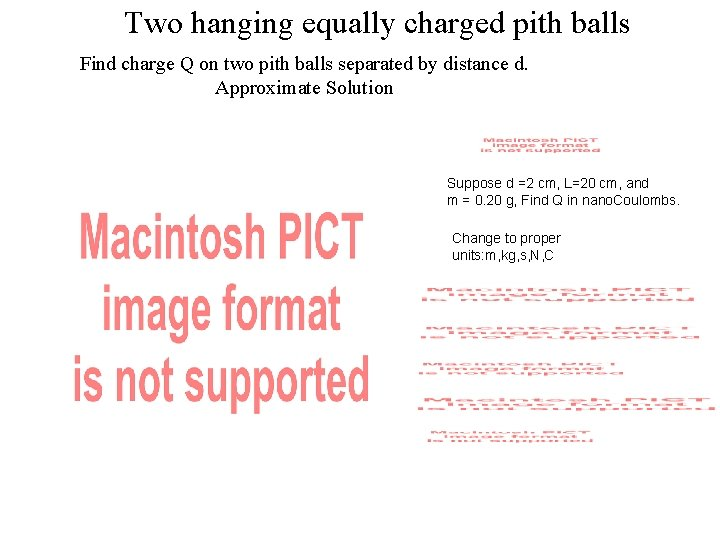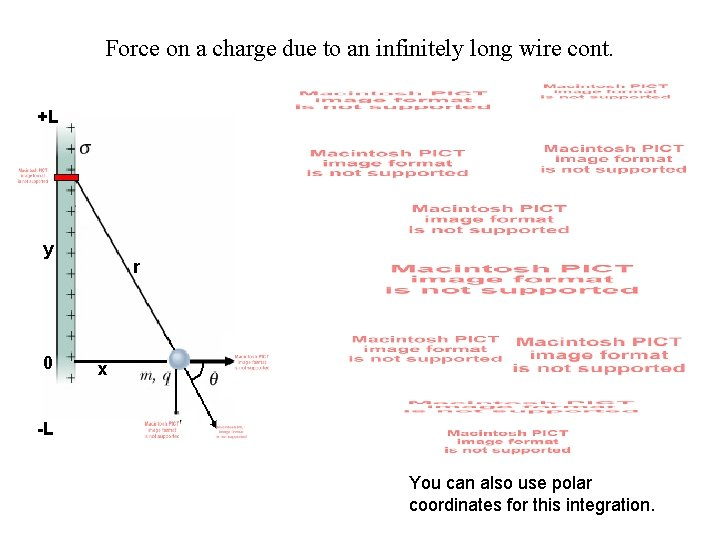# Coulombs Law Two point Charges What is the

• Slides: 9Coulombs Law Two point Charges • What is the force between two positive charges each 1 nano. Coulomb 1 cm apart in a typical demo? q 1 r q 2 1 n. C 1 cm 1 n. C Repulsion (equivalent to a weight of something with a mass of 10 -5 kg = 10 -2 gm or 10 mg - long strand of hair)Coulombs Law Two Pennies What is the force between two 3 gm pennies one meter apart if we remove all the electrons from the copper atoms? (Modeling) The force is The atom Cu has 29 protons and a 3 gm penny has = The total charge is What is their acceleration as they separate?Principle of Superposition Three Charges in a Line • In the previous example we tacitly assumed that the forces between nuclei simply added and did not interfere with each other. That is the force between two nuclei in each penny is the same as if all the others were not there. This idea is correct and is referred to as the Principle of Superposition. • Example of charges in a line x 1 2 3 – Three charges lie on the x axis: q 1=+25 n. C at the origin, q 2= -12 n. C at x =2 m, q 3=+18 n. C at x=3 m. What is the net force on q 1? We simply add the two forces keeping track of their directions. Let a positive force be one in the + x direction.Two hanging equally charged pith balls Find charge Q on two pith balls separated by distance d. Approximate Solution Suppose d =2 cm, L=20 cm, and m = 0. 20 g, Find Q in nano. Coulombs. Change to proper units: m, kg, s, N, CExact SolutionForce on a charge due to an infinitely long wire. Charge Q is uniformly distributed on the infinite wire with charge density l. We choose the Y-axis through the line charge and the X-axis through the point charge. Now, because the line charge is infinite and straight (on Y-axis), we can assume the X-axis is the mid-point of the infinite wire. Then by Coulomb’s law for the small line segment dy +L Resolving the forces into its components: y 0 -L r x As it is can be seen that the y-component of the force exerted by the top half of the infinite wire will cancel the y-component force exerted by the bottom half of the infinite wire.Force on a charge due to an infinitely long wire cont. +L y 0 r x -L You can also use polar coordinates for this integration.